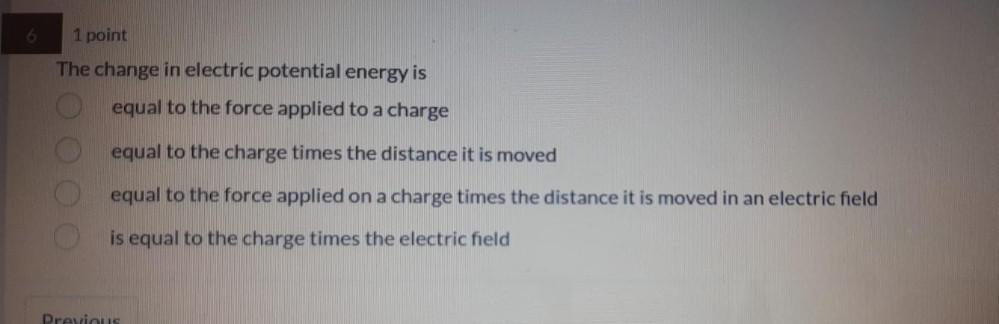Question:

# 1 point The change in electric potential energy is equal to the force applied to a charge equal to the charge times the distance1 point The change in electric potential energy is equal to the force applied to a charge equal to the charge times the distance it is moved equal to the force applied on a charge times the distance it is moved in an electric field is equal to the charge times the electric field Previous